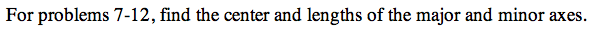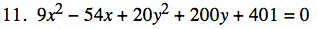13E-11.

### Missing problem for Book PCT => Chapter Ch13 => Lesson 13.1.2 => Problem 13E-11

Created from orphaned homework help problemRefer to Example 3 to assist you with this problem.

Complete the square to rewrite this equation in Standard Form.

$9\left(\textit{x}^2-6\textit{x}+9\right)+20\left(\textit{y}^2+10\textit{y}+25\right)=-401+9\left(9\right)+20\left(25\right)$